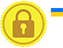# Basic Arithmetic Operators in javascript

In javascript we can do ordinary arithmetic operation such as addition, subtraction, multiplication, division.

Symbol Name Description Example
`+` Plus Used for addition operation. Try Yourself
`-` Minus Used for subtraction operation Try Yourself
`*` Multiply Used for multiplication operation. Try Yourself
`/` Divide Used for division operation Try Yourself

### Example

Try Yourself

In this example two variables a and b are created and values are assigned(30,10). These two values are added using + symbol and the resultant value is stored in variable c and displayed.

# Subtraction operation in javascript

### Example

Try Yourself

In this example two variables a and b are created and values are assigned(30,10). These two values are subtracted using - symbol and the resultant value is stored in variable c and displayed.

# Multiplication operation in javascript

### Example

Try Yourself

In this example two variables a and b are created and values are assigned(30,10). These two values are multiplied using * symbol and the resultant value is stored in variable c and displayed.

# Division operation in javascript

### Example

Try Yourself

In this example two variables a and b are created and values are assigned(30,10). These two values are divided using / symbol and the resultant value is stored in variable c and displayed.